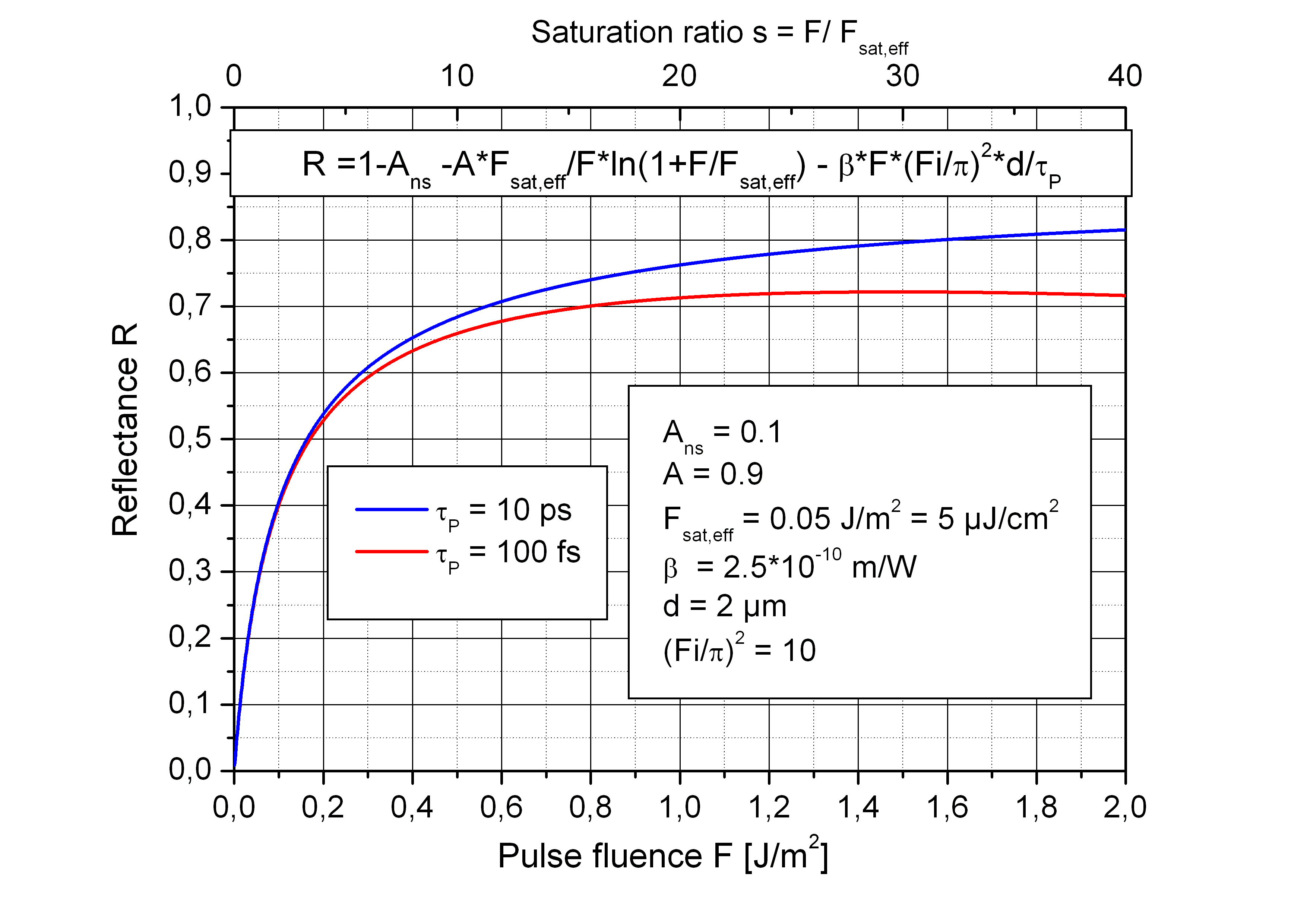> Energy band gap> GaAs | AlxGa1-xAs | InxGa1-xAs
> Refractive index > GaAs | AlAs | AlxGa1-xAs | InxGa1-xAs
> Devices > Bragg mirror | SAM | RSAM | SA | SANOS | SOC | Microchip | PCA
> Device application > Papers | Patents | FAQs## RSAM - Resonant saturable absorber mirror

##### RSAM construction

The resonant saturable absorber mirror (RSAM) is a similar device as a saturable absorber mirror (SAM), but has a larger saturable absorption, a smaller bandwidth and a lower saturation fluence. The RSAM is designed as a resonant Gires–Tournois interferometer with absorber layers positioned at the antinodes of the optical field inside the resonator cavity.
The RSAM is a nonlinear optical device, having a very low reflectance for week optical signals like noise and a high reflectance for high power signals like optical pulses. Optical pulses saturate the absorber material inside the resonant cavity of the RSAM. Due to the short recovery time of the absorber material the RSAM blocks immediately after the reflected pulse the optical noise floor.
Important parameters of a RSAM are resonance wavelength, bandwidth and saturation fluence.

RSAM applications

• Optical noise suppression, for example after an EDFA or a pulse picker → SANOS
• Opto-optical wavelength conversion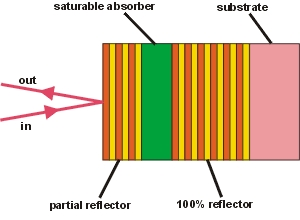Resonance wavelength

Resonance wavelength

Dependence of the resonance wavelength on the angle of incidence

The resonance wavelength λ of the Gires–Tournois interferometer depends on the angle of incidence φ and is give bywith
• λ - resonance wavelength of the interferometer
• n - refractive index of the absorbing spacer layer
• d - thickness of the absorbing spacer layer
• m - order of the resonance; m = 1, 2, 3, ....
• φ - angle of incidence on the RSAM

In case of a perpendicular beam incidence φ = 0 the first order resonance wavelength is λ = 2·n·d.

The shift of the resonance to shorter wavelengths with increasing angle of incidence φ is shown in the figure right. The resonance wavelength at perpendicular incidence is λ0 = 1064 nm and the refractive inder n = 3.1.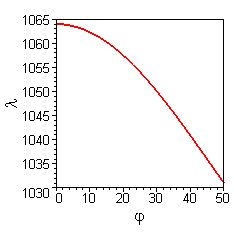Resonance wavelength for parallel polarized light
at different angles of incidence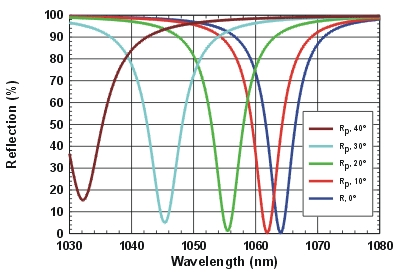Resonance wavelength for perpendicular polarized light
at different angles of incidence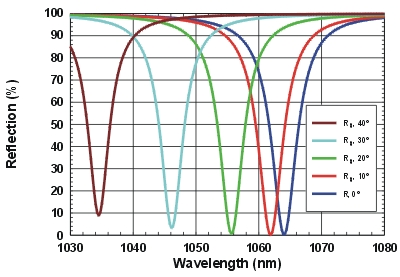Saturation behaviour

Saturation behaviour

Dependence of the RSAM reflectance on pulse fluence

• Because a non-saturated RSAM has zero reflectance at resonance wavelength low intensity signals at this wavelength are absorbed and not reflected.
• The first photons of an optical pulse are absorbed. They saturate partially the absorber.
• The RSAM reflectance at the resonance increases with increasing absorber saturation.
• The appropriate measure for the saturation is the pulse fluence F ~ I · τ (I - pulse intensity, τ - pulse duration).
• The RSAM can only suppress low signals with the resonance wavelength. All signals outside the resonance are reflected dependent on the spectral distance from resonance.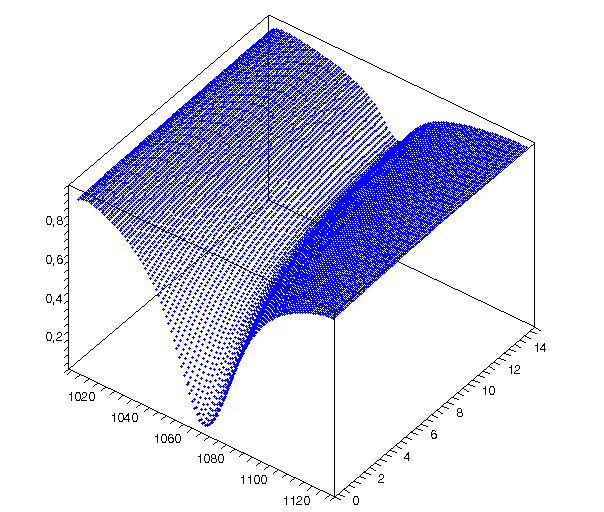Reflectance of an RSAM as a fucntion of wavelength (in nm) and pulse fluence F (in J/m2). The resonance wavelength is λ = 1064 nm.

Influence of temperature

Influence of temperature

There is also a temperature influence on the resonance wavelength λ. The temperature dependency of the optical thickness n·d of the absorbing spacer layer, which governs the resonance wavelengthλ is mainly determined by the refractive index. The influence of the thermal expansion of the layer thickness is negligible.
The change of the resonance wavelength λ with the temperature can be calculated by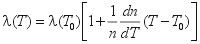• λ(T) - resonance wavelength at temperature T
• λ(T0) - resonance wavelength at reference temperature T0
• 1/n*dn/dT - ~ 7x10-5K-1, temperature coefficient of refractive index
• T0 - reference temperature
• T - working temperature.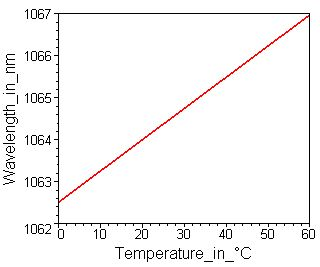Bandwidth

Bandwidth

The bandwidth Δλ of the interferometer resonance dip is gouverned by the round trip loss l of the wave inside the cavity and can be estimated in case of small losses l << 1 by

Δλ = λ · (1-√Rf + A)/ (m · π) = λ · l / (m · π)

• Δλ - bandwidth FWHM (full width at half maximum)
• λ - resonance wavelength of the interferometer
• Rf - reflectance of the front mirror (back mirror reflectance Rb = 1)
• A - single pass absorptance of the spacer layer
• m - order of the resonance; m = 1, 2, 3, ....
• l -round trip loss: 1 - √Rf + A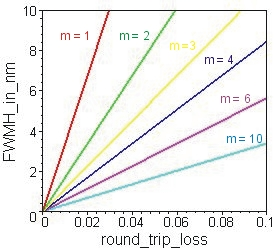RSAM, impedance matched at λ = 1064 nm with

• Front mirror reflectance Rf = 0.72
• Unsaturated absorptance A = 0.0016 .. 0.16
• Resonance order m = 4.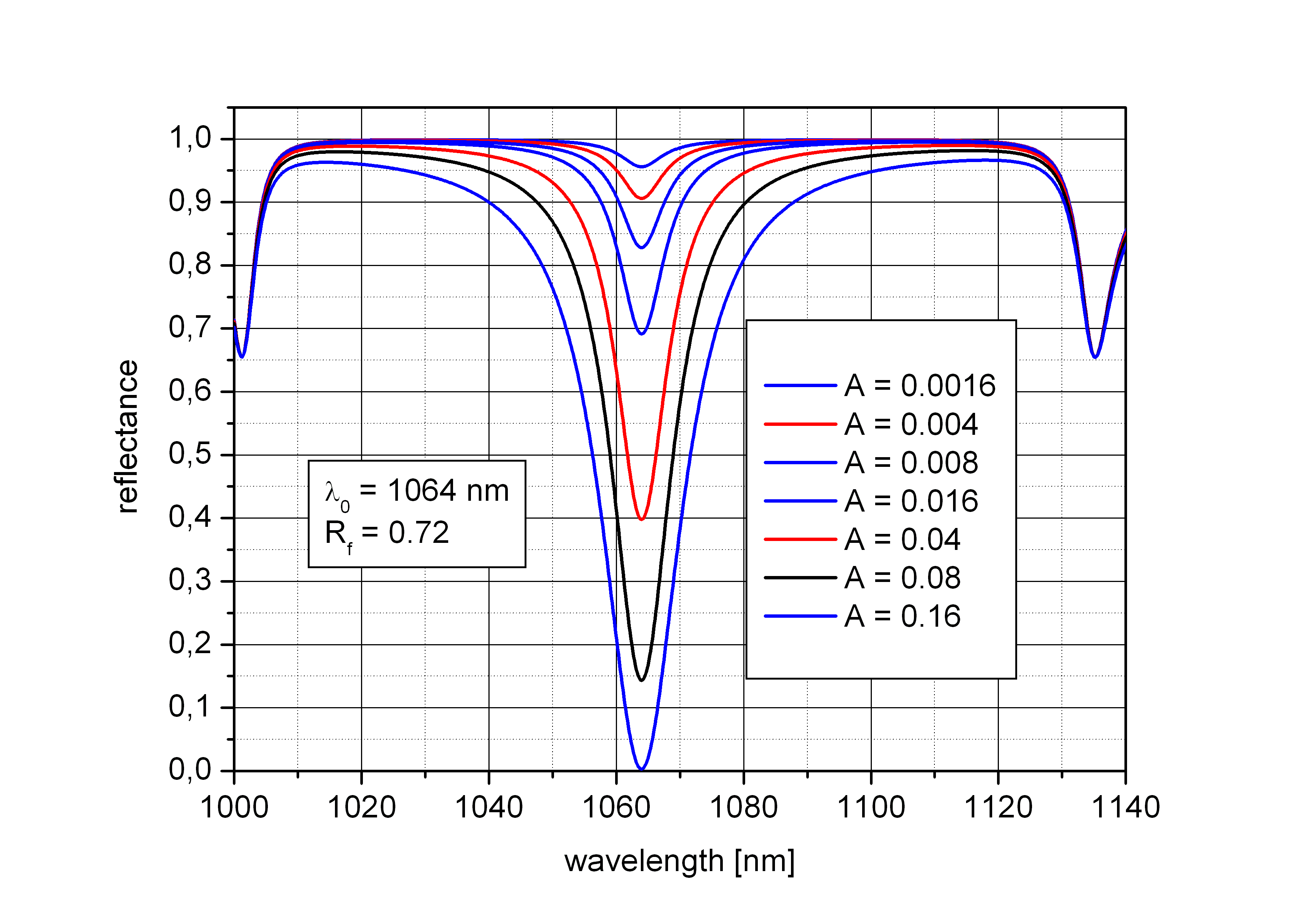Saturation intensity

Saturation intensity

The RSAM is a strong nonlinear optical device. The absorptance A of the absorber layer and the reflectance R of the RSAM depend on the incoming light intensity I. Due to the resonance condition of the Gires–Tournois interferometer at the working wavelength the effective saturation intensity Isat,eff of the device shifts by a factor of about (π/F)2 (F - finesse of the Gires–Tournois interferometer) to lower values in relation to the intrinsic material value Isat, which is valid for non-resonant saturable absorber mirrors (SAM).
The absorptance A of a RSAM with a not too small finesse Fi > 10 can be estimated by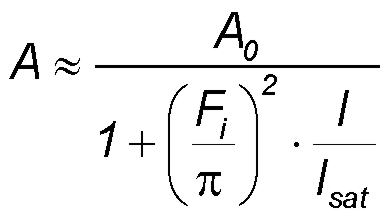with

• A - absorptance
• A0 - small signal absorptance (saturable absorption)
• I - light intensity (measured in W/m2)
• Isat - intrinsic material saturation intensity
• Fi - finesse of RSAM.

The effective saturation fluence Fsat,eff  of a RSAM can be estimated using the relaxation time τ and the effective saturation intensity Isat,eff

Fsat,eff ≈ Isat,eff·τ ≈ (π/Fi)2· Isat·τ

with

• Fsat,eff - effective saturation fluence of the RSAM
• Isat,eff - effective saturation intensity of the RSAM
• τ - relaxation time of the absorber material

With typical values for a non-resonant SAM

• Isat = 10 MW/cm2
• τ = 10 ps
• Fsat = 100 µJ/cm2

the relevant parameters for a RSAM with a finesse F = 20 can be estimated to

• Isat,eff = 250 kW/cm2
• Fsat,eff = 2.5 µJ/cm2

The effective saturation values can be decreased to very low values on the expense of a small spectral bandwidth.

Intensity dependent reflectance

Intensity dependent reflectance

Despite the effective saturation intensity Isat,eff of a RSAM is smaller then the saturation intensity Isat of a SAM the heat production in the absorber layer by using a continuous large signal would be significant. Therefore also the RSAM is used typically with pulsed signals of pulse duration τ ≤ 50 ps.
The saturation of a RSAM can be described in the same way as the saturation of a SAM.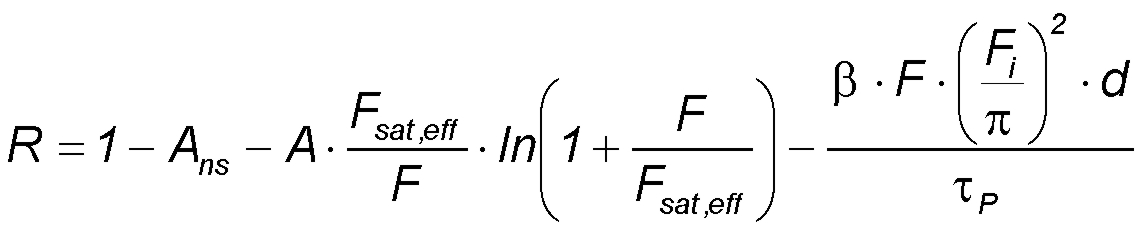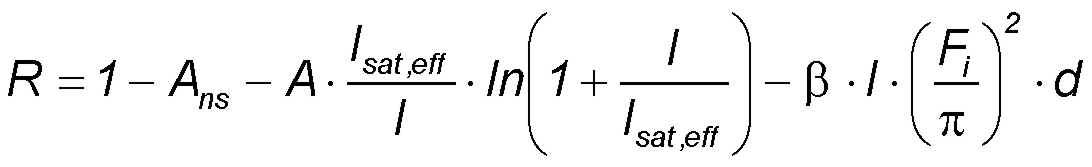with

• A - saturable absorption
• Ans - non-saturable absorption
• F - pulse fluence
• Fsat,eff = Fsat·(π/Fi)2- effective saturation fluence
• I = F/τP - pulse Intensity
• Isat,eff - effective saturation intensity
• β - two-photon absorption coefficient
• d - absorber layer thickness
• τP - pulse duration.

The non-saturable absorption Ans depends mainly on the relaxation time of the absorber layer. With shorter relaxation time increases Ans. The two photon absorption increases with shorter pulses.